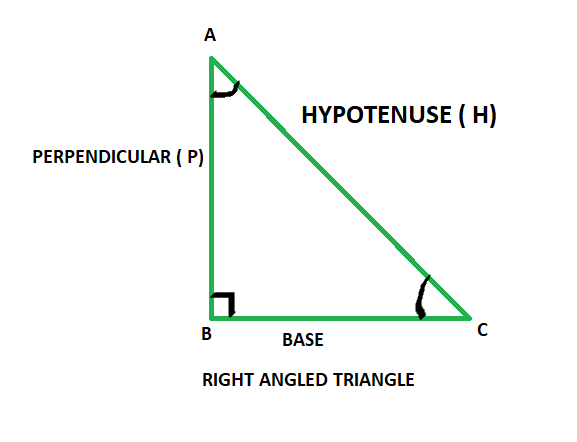# How to find the value of tan 150°?

• Last Updated : 01 Jan, 2022

Trigonometry is a discipline of mathematics that studies the relationships between the lengths of the sides and angles of a right-angled triangle. Trigonometric functions, also known as goniometric functions, angle functions, or circular functions, are functions that establish the relationship between an angle to the ratio of two of the sides of a right-angled triangle. The six main trigonometric functions are sine, cosine, tangent, cotangent, secant, and cosecant.

Angles defined by the ratios of trigonometric functions are known as trigonometry angles. Trigonometric angles represent trigonometric functions. The value of the angle can be anywhere between 0-360°.As given in the above figure in a right-angled triangle:

• Hypotenuse: The side opposite to the right angle is the hypotenuse, It is the longest side in a right-angled triangle and opposite to the 90° angle.
• Base: The side on which angle C lies is known as the base.
• Perpendicular: It is the side opposite to angle C in consideration.

Trigonometric Functions

Trigonometry has 6 basic trigonometric functions, they are sine, cosine, tangent, cosecant, secant, and cotangent. Now let’s look into the trigonometric functions. The six trigonometric functions are as follows,

sine: It is defined as the ratio of perpendicular and hypotenuse and It is represented as sin θ

cosine: It is defined as the ratio of base and hypotenuse and it is represented as cos θ

tangent: It is defined as the ratio of sine and cosine of an angle. Thus the definition of tangent comes out to be the ratio of perpendicular and base and is represented as tan θ

cosecant: It is the reciprocal of sin θ and is represented as cosec θ.

secant: It is the reciprocal of cos θ and is represented as sec θ.

cotangent: It is the reciprocal of tan θ and is represented as cot θ.

Trigonometric Identities of Complementary and Supplementary Angles

• Complementary Angles: Pair of angles whose sum is equal to 90°
• Supplementary Angles: Pair of angles whose sum is equal to 180°

Identities of Complementary angles are

sin (90° – θ) = cos θ

cos (90° – θ) = sin θ

tan (90° – θ) = cot θ

cot (90° – θ) = tan θ

sec (90° – θ) = cosec θ

cosec (90° – θ) = sec θ

Identities of supplementary angles

sin (180° – θ)  = sin θ

cos (180° – θ) = – cos θ

tan (180° – θ) =  – tan θ

cot  (180° – θ) = – cot θ

sec (180° – θ) = – sec θ

cosec (180° – θ) = – cosec θ

### How to find the value of tan 150°?

Solution:

Since, we know that tan is positive in the 1st and 3rd Quadrant,

here, 150° lies in the 2nd Quadrant, then

By the Trigonometric Identity of Supplementary Angles,

We know that tan (180° – θ)  = tan θ

Hence,

tan 150° = tan(180° – 30°)

= – tan 30°                 {As given by Identity}

= – 1/√3

### Similar Questions

Question 1: What is the exact value of sec 150°?

Solution:

Here sec is positive only in the 1st and 4th Quadrant.

150° lies in the 2nd Quadrant.

Therefore

sec (180° – θ) = – sec θ

sec (150°) = sec (180° – 30°)

sec (150°) = – sec (30°)

sec (150°) = – 2

So the exact value of sec 150° is 2

Question 2: What is the Exact value of cot 150°?

Solution:

Here cot is positive only in 1st and 3rd Quadrant.

Therefore cot(180° – θ) = – cot θ

cot(150°) =  cot(180° – 30°)

cot(150°) = – cot(30°)

cot (150°) = -√3      { as per the trigonometry value table }

So the exact value of cot 150° is -√3

My Personal Notes arrow_drop_up
Recommended Articles
Page :